# Query-based Instance Discrimination Network for Relational Triple Extraction

## Basic Idea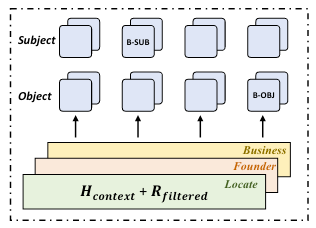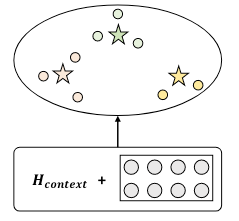## QIDN

QIDN的概览模型图如下: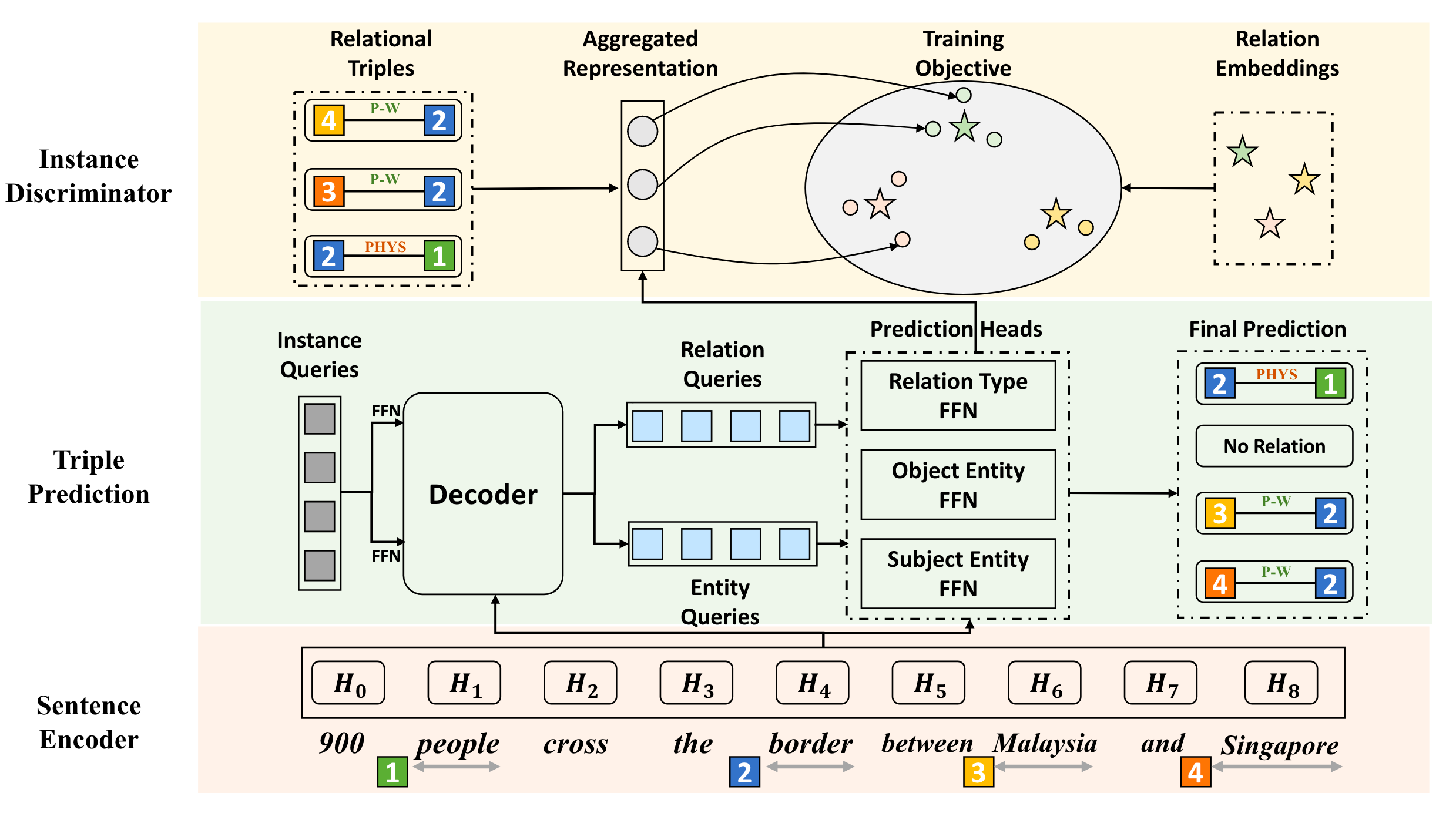### Sentence Encoder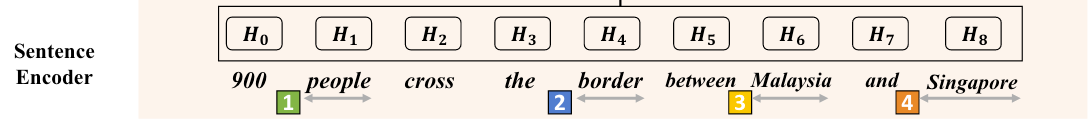### Triple Prediction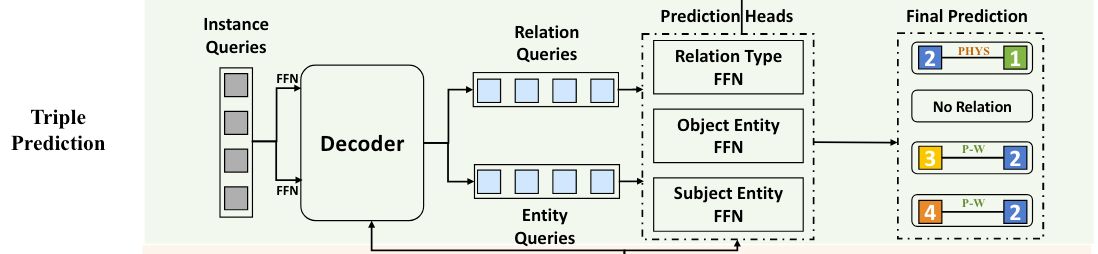DETR最早被用于目标检测, 每个Query都对应了图像中的一个目标(Bounding Box和物体类别). 与之类似的, 在QIDN中, 每个Query对应着一个关系三元组.

#### Transformer - based Decoder

Decoder是由$L$ 层的Transformer Decoder的堆叠, 其中Attention计算方式如下:

$$\text { Attention }(Q, K, V)=\operatorname{softmax}\left(\frac{Q K^T}{\sqrt{d_k}}\right) V$$

Transformer Decoder的堆叠可以记为:

$$\operatorname{Decoder}(Q, H^\prime) = \operatorname{Attention}(Q, H^\prime, H^\prime)$$

$$H_{\mathrm{i}}^{\text {span }}=\left[H_{\text {start }(\mathrm{i})} ; H_{\mathrm{end}(\mathrm{i})} ; \phi\left(s_{\mathrm{i}}\right)\right]$$

$[;]$ 为拼接操作, $H_{\text {start }(\mathrm{i})} , H_{\mathrm{end}(\mathrm{i})}$ 分别为起始和结束Token的表示, $\phi(s_i)$ 为NER中Span based方法常用的长度Embedding, 可以加入一些Span的长度信息. 最终Span Level的表示为$H^{span} \in \mathbb{R}^{n_s \times d}$.

$$\left[Q_r ; Q_e\right]=\operatorname{Decoder}\left(\left[Q W_r ; Q W_e\right], H^{\text {span }}\right)$$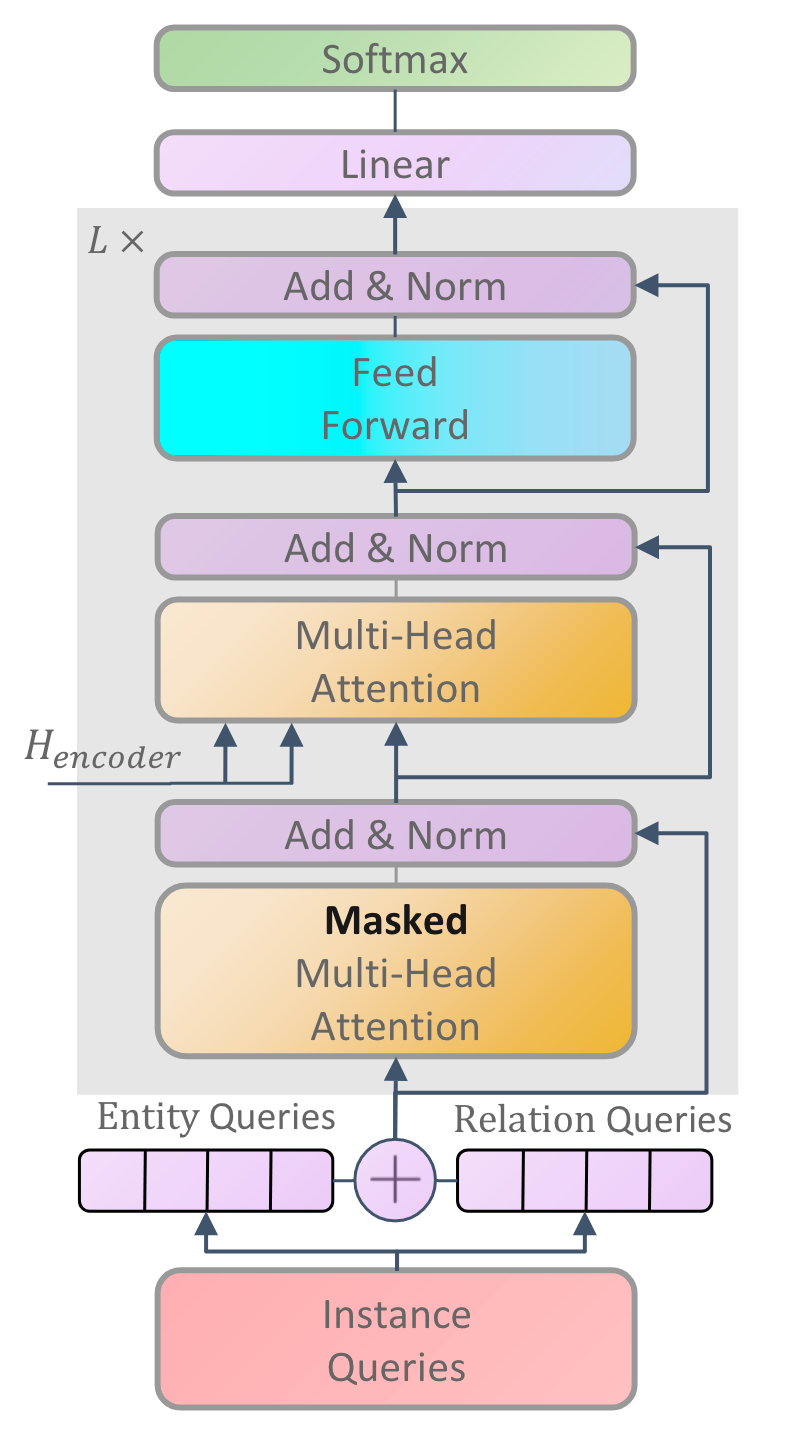$$P_{i c}^t=\frac{\exp \left(Q_r^i W_t^c+b_t^c\right)}{\sum_{c^{\prime}}^{\left|\mathcal{Y}_r\right|} \exp \left(Q_r^i W_t^{c^{\prime}}+b_t^{c^{\prime}}\right)}$$

\begin{aligned} E_\delta=Q_e W_{\delta}\\ H_s=H W_s \end{aligned}

$$S\left(\mathbf{v}_i, \mathbf{v}_j\right)=\frac{\mathbf{v}_i}{\left|\mathbf{v}_i\right|} \cdot \frac{\mathbf{v}_j}{\left|\mathbf{v}_j\right|}$$

$$P_{i j}^\delta=\frac{\exp S\left(E_\delta^i, H_s^j\right)}{\sum_{j^{\prime}}^n \exp S\left(E_\delta^i, H_s^{j^{\prime}}\right)}$$

### Instance Discriminator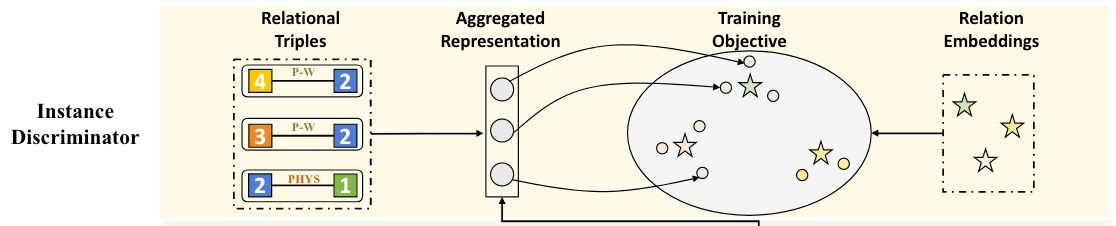#### Aggregation

$$\mathbf{v}=Q_r W+\sum_{\delta \in \mathcal{C}} E_\delta$$

#### Training Objective

1. 对于三元组实例和实例之间, 应满足同关系内更近, 不同关系的更远.
2. 对于三元组实例和关系之间, 应满足三元组和自身关系对应的关系嵌入更近.

$$\mathcal{L}_{\mathrm{ins}}=-\sum_c \sum_{i, j} \log \frac{\exp S\left(\mathbf{v}_i^c, \mathbf{v}_j^c\right)}{\sum_{c^{\prime}, j^{\prime}} \exp S\left(\mathbf{v}_i^c, \mathbf{v}_{j^{\prime}}^{c \prime}\right)}$$

$$\mathcal{L}_{\mathrm{cls}}=-\sum_{i, c} \log \frac{\exp S\left(\mathbf{v}_i^c, \mathbf{r}_c\right)}{\sum_{c^{\prime}} \exp S\left(\mathbf{v}_i^c, \mathbf{r}_{c^{\prime}}\right)}$$

### Training and Inference

#### Training

$$\mathcal{L}_{\text {tri }}=-\sum_{i=1}^M\left(\log P_{\sigma(i)}^t+\sum_{\delta \in \mathcal{C}} \log P_{\sigma(i)}^\delta\right)$$

$M$ 为Query的数量, $\sigma$ 为最优匹配的三元组.

$$\mathcal{L} = \mathcal{L}_{tri} + \mathcal{L}_{ins} + \mathcal{L}_{cls}$$

#### Inference

• 三元组可以由Instance Query$\mathcal{Y}_i=\left(\mathcal{Y}_i^t, \mathcal{Y}_i^\delta\right), \delta \in \mathcal{C}$ 得到.
• 关系类型预测可以由$\mathcal{Y}_i^t=\arg \max _c\left(P_{i c}^t\right)$ 得到.
• 头尾实体的左右边界可以由$\mathcal{Y}_i^\delta=\arg \max _k\left(P_{i k}^\delta\right)$ 得到.
• 预测类型为$\varnothing$ 的三元组直接被滤去.

## Experiments

### Dataset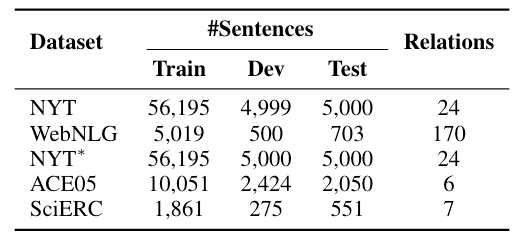### Overall Performance

QIDN在RTE三个数据集NYT, WebNLG*, NYT* 上结果如下: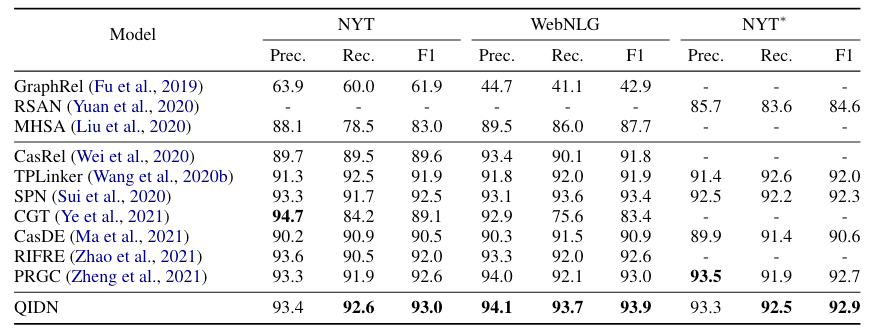QIDN相较于作者给出的Baseline有非常大的进步, 这个性能其实也挺能打的.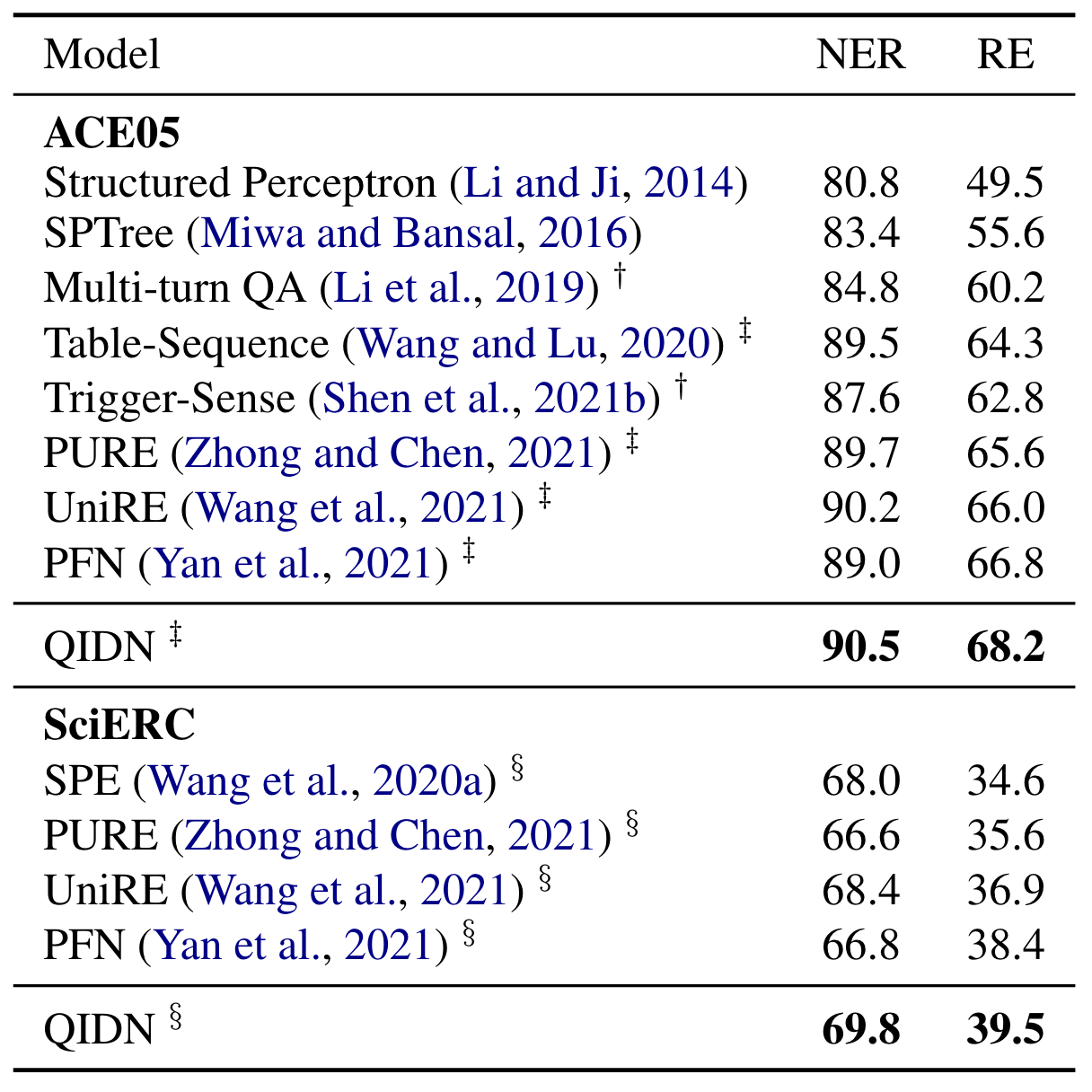### Analysis on Complex Scenarios

QIDN在NYT*和WebNLG*复杂场景表现如下: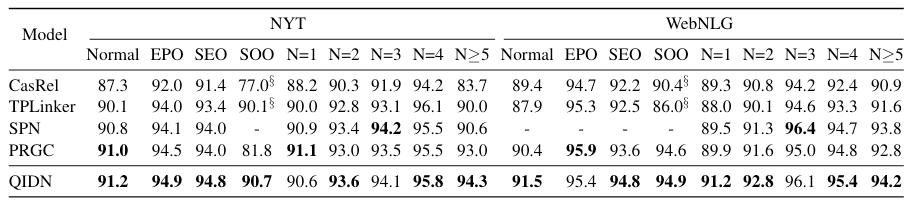### Ablation Study

• w/o $H_{span}$: 将Span Level表示替换为Token Level表示.
• w/o $Q_e, Q_r$: 去掉对实体和关系Query的特化, 统一用同一种Query来代替.
• w/o $\mathcal{L}_{ins}$: 去掉Instance Pair之间的对比学习Loss.
• w/o $\mathcal{L}_{cls}$: 去掉Instance和Relation Embedding之间的对比学习Loss.
• w/o $\mathcal{L}_{ins}, \mathcal{L}_{cls}$: 去掉所有对比学习Loss.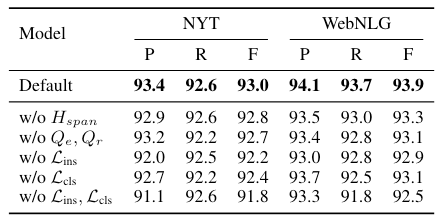### Topology of Relations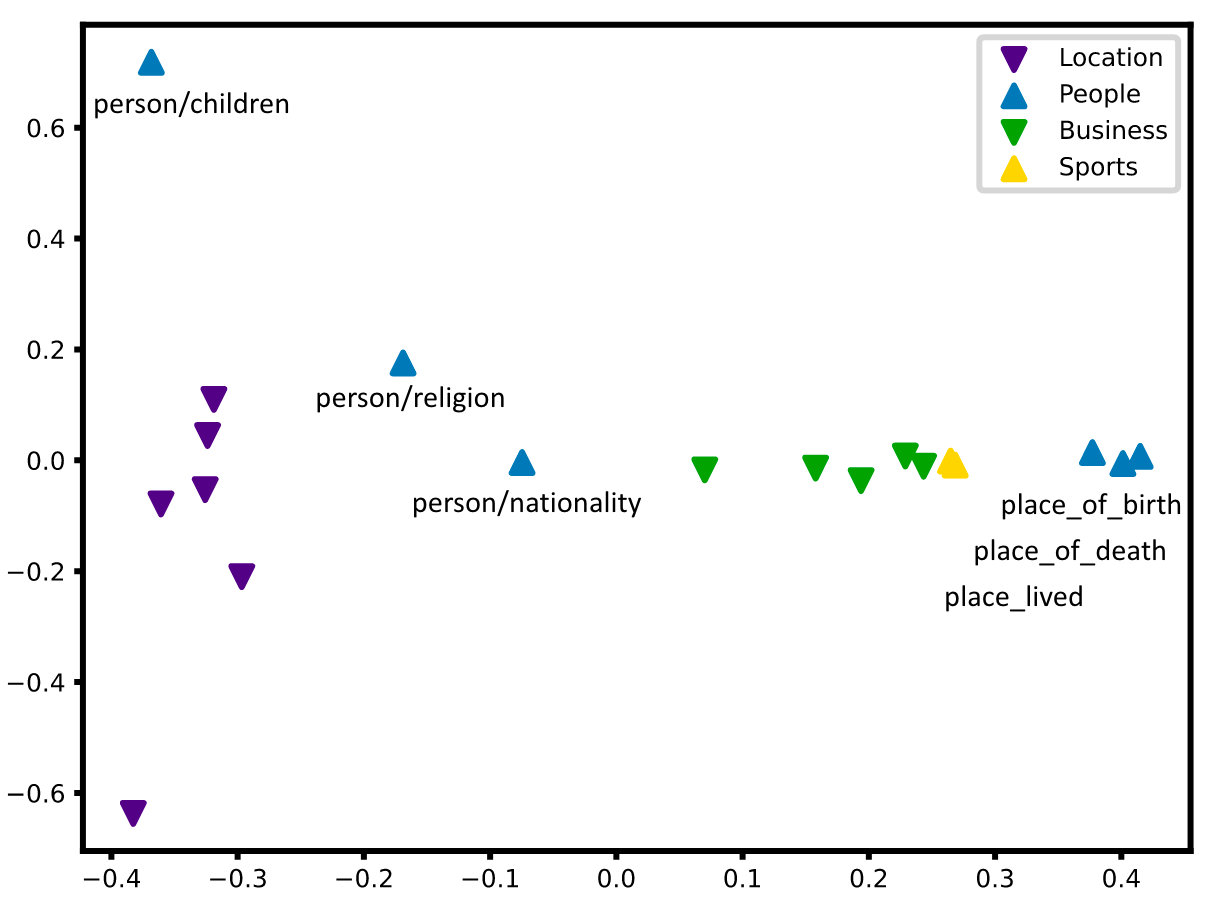### Error Analysis

• NER: ECE(Entity Classification Error), ELE(Entity Localtion Error).
• RE: RCE(Relation Classification Error), PCE(Entity-Pair Classification Error), PLE(Entity-Pair Location Error).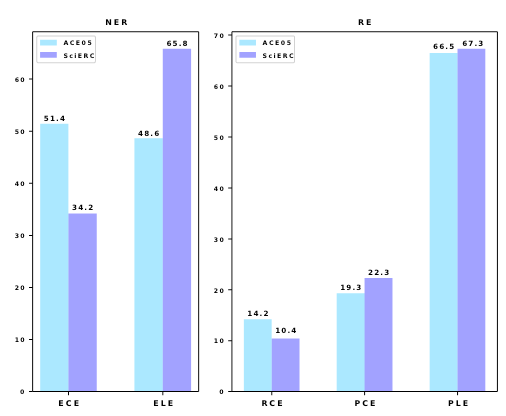## Summary

QIDN用Query based方法, 从Instance Level的角度解决了RE任务. 将Instance Query拆分为Relation QueryEntity Query两个branch从而将Query对应的三元组抽取出来, 同时在空间中用对比学习约束三元组, 使得三元组之间存在全局关联, 并捕获了关系的语义信息.

上一篇Large Model并行优化
Large Model并行优化为什么要并行优化?大就是好, 虽然丛2019年人们的认识普遍就是大就是好, 这个概念在当今依然没有被改变, 只是有了更深刻的认识. 所以, 为什么要并行? 虽然大就是好, 模型太大显存吃不消(空间)
2023-06-01UniRel: Unified Representation and Interaction for Joint Relational Triple Extraction
UniRel: Unified Representation and Interaction for Joint Relational Triple Extraction本文是论文UniRel: Unified Representation
2023-01-03
目录